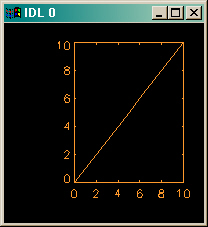Closest Match in Color Table

QUESTION: Suppose I have a color, say yellow. How do I find the index of the color that is the closest match to yellow in the current color table?ANSWER: The easiest way to find the closest match in the color table is to make this a 3D distance problem and minimize the distance from the color to the color table possibilities. This is easily done in IDL if you remember that all colors in IDL are represented as RGB triples.

For example, the color yellow can be represented in IDL as a three-element RGB array, like this:

IDL> yellow = [255, 255, 0]

The idea is to simply minimize the difference between these three values and the sets of other three values, as represented in the current color table. To load a blue-to-red color table, get the current color table values, and put them into the variables R, G, and B,we do this:

IDL> TVLCT, R, G, B, /Get

Next, we create a distance variable, and then find the index in which this distance is a minimum. To prevent overflow, however, it is essential that the R, G, and B variables be cast to LONG data type. The code looks like this:

IDL> R = Long(R)
IDL> G = Long(G)
IDL> B = Long(B)
IDL> distance = (R-yellow)^2 + (G-yellow)^2 + (B-yellow)^2
IDL> index = Where(distance EQ Min(distance))

To see that this worked, draw a plot with this color index. Note that we need to make the index a scalar value (it returns from the Where function as a vector).

IDL> index = index
IDL> Plot, Findgen(11), Color=index
IDL> Print, R[index], G[index], B[index]
255   255   0

You see the results of this command in the figure below. Note that if a yellow color does not exist in the color table, you will still return the color that is the closest "match" to a yellow color.

You should be aware, however, that this may not look anything like yellow. For example, here are the results when color table 3, a red-temperature color table is used instead of the blue-to-red color table above.

IDL> Print, R[index], G[index], B[index]
255   153   43